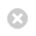Close

UCL Astrophysics Group

Home# Modelling

The Atmospheric Physics Laboratory has been developing various models of the upper atmosphere over the past two decades.

We widely collaborate with other academic institutions and professional bodies in order to further our understanding of upper atmospheric phenomena.

At any point in the atmosphere, it is possible to calculate how much energy is being received or lost, due to processes such as solar heating, diffusion, wind transport, and re-emission of radiation. From this net value, the amount that local temperature would change, in for example 60 seconds, can be determined. If we add this change to the current value of temperature, we get the temperature in 60 seconds time. This is basically how our models calculate temperature, winds, and composition; by calculating the rate of change due to physical processes that occur in the real atmosphere, and stepping forward the current value accordingly every 60 seconds of model time. This is done on a global grid with points in latitude, longitude, and pressure height.

The most challenging aspect of this technique is the representation within a computer code of physical processes that occur in the real world. They are complex, made more so by the fact that temperature, winds and composition all interact in convoluted ways. Much of the physics is poorly understood, which is why our models are so useful in attempting to determine how the real atmosphere works. Another important issue is the staggering number of calculations required to run simulations. To cope with this we often run our suite of models on various supercomputers provided by University College London.Example of global temperature variation using our CMAT2 model